# OECD Nuclear power plants data analysis 4 - Getting confidence interval for one proportion using R infer packageUnsplashMaarten van den Heuvelが撮影した写真

This post is following of the above post.

In this post, I will get confidence interval for one proportion. In this case, number of nuclear power plants in Japan / number of nuclear power plants on earth.

First, I make a new dataframe to calculate the proportion.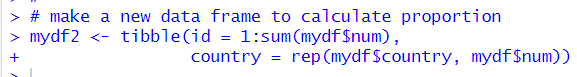Let's see data structure of mydf2.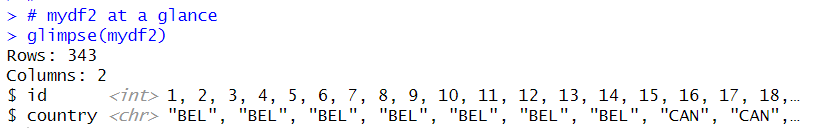Then, I add a new variable to indicate whether JPN or not.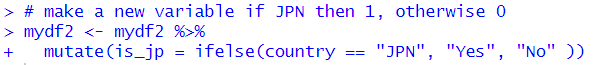I make bar chart to show how many nuclear power plants in Japan.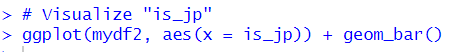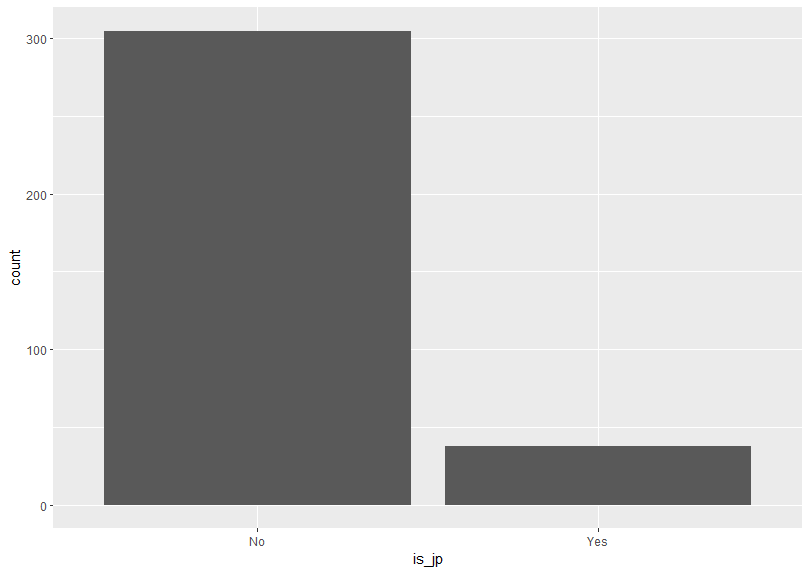So, I see there are much less nuclear power plants in Japan compare to other countries total.

I calculate the proportion, the number of nuclear power plants in Japan / total number of nuclear power plants on the earth.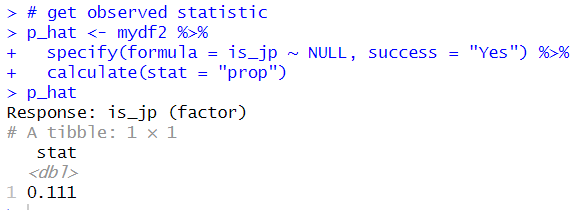Above is using infer package specify() function and calculate() function.

Simple way is below.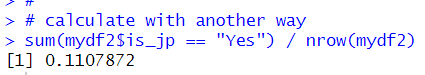So, I know 0.111( or 0.1107872) is the proportion.

I would like to calculate confidence interval for the proprtion, if it is random variable.

I make bootstrap distribution of it.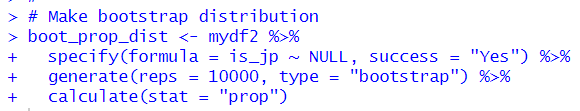Let's visualize this distribution.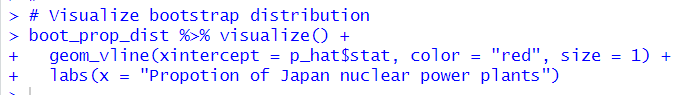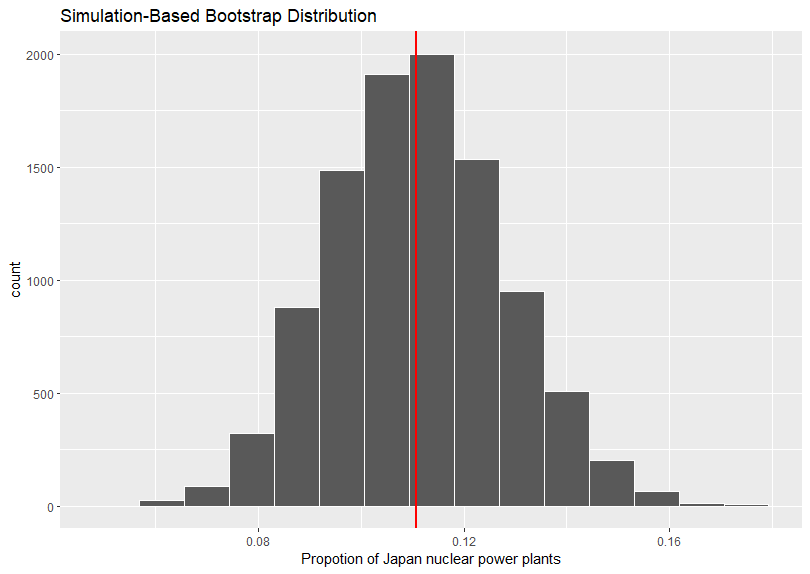The vertical red line is observed proportion, 0.111.

Let's get confidence interval at 95% level.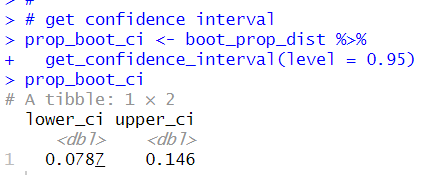The confidence interval is from 0.0782 to 0.146. It means that if I go to another multiverse world, I am 95% confident that Japan has 0.0782 to 0.146 proportion of nuclear power plants in the world.

Let's visualize this confidence interval.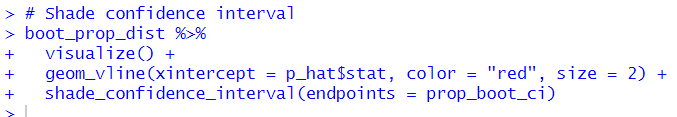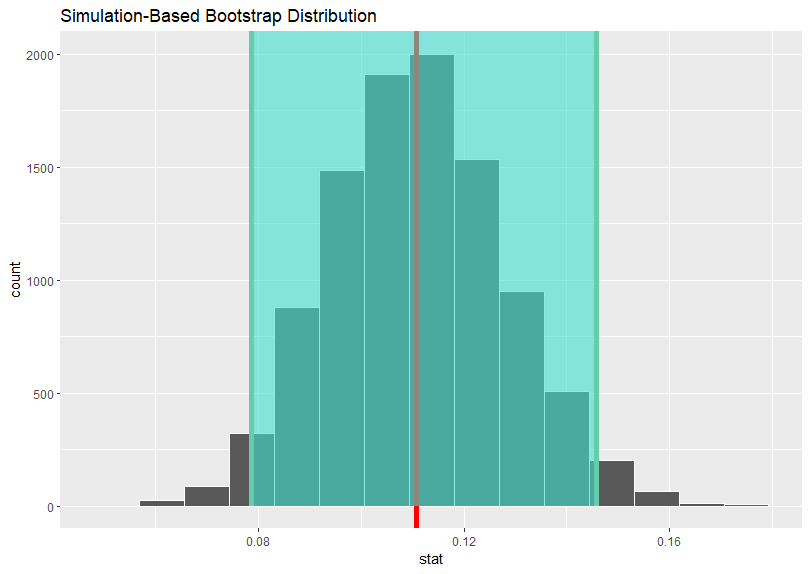That's it. Thank you!

Next post is